Worksheet Question: Understanding Elementary Shapes

# Worksheet Question: Understanding Elementary Shapes - Notes | Study Worksheets for Class 6 - Class 6

 1 Crore+ students have signed up on EduRev. Have you?

Ques 1: Match the column B with A and C:

 A B C (a) Straight angle (i) An angle whose measure is less than 90°. (f) One fourth of a revolution. (b) Right angle (ii) An angle whose measure is between 90° and 180°. (g) Half of a revolution. (c) Acute angle (iii) An angle whose measure is 180°. (h) More than half a revolution (d) Obtuse angle (iv) An angle whose measure is 90°. (i) Less than one-fourth of a revolution. (e) Reflex angle (v) An angle whose measure is between 180° and 360°. (j) Between ¼ and ½ of a revolution.

For example (a) → (iii) → (g)
Ans: (a) → (iii) → (g)
(b) → (iv) →  (f)
(c) → (i) →  (i)
(d) → (ii) →  (j)
(e) → (v) →  (h)

Ques 2: A sailor is rowing a boat due north. In which direction will he be rowing if he turns it through (i) a straight angle (ii) a complete angle
Ans: (i) South
(ii) North

Ques 3: You are standing in a class-room facing north. In what direction will you be facing after making a quarter turn in clockwise direction?
Ans: East

Ques 4: Write five capital letters of the English alphabet which show perpendicular lines/line segments.
Ans: E, F, H, I, J, L, T (any five)

Ques 5: Identify the following triangles according to the measurement of sides and angles given. Also mention the type of triangle: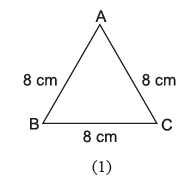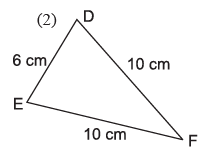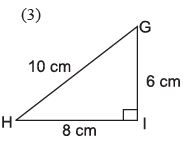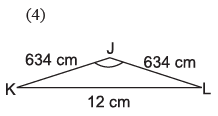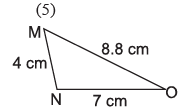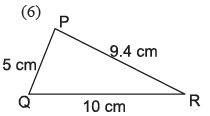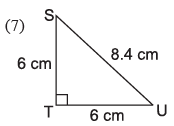(e.g. (1) ∆ABC is an equilateral triangle and acute angled triangle.
Ans: (1) ∆ABC: Equilateral and acute angled triangle
(2) ∆DEF: Isosceles triangle and acute angled triangle
(3) ∆GIH: Scalene triangle and right angled triangle
(4) ∆JKL: Isosceles triangle and obtuse angled triangle
(5) ∆MNO: Scalene triangle and obtuse angled triangle
(6) ∆PQR: Scalene triangle and acute angled triangle
(7) ∆STV: Isosceles triangle and right angled triangle

Ques 6: Fill in the blanks:
(a) Each angle of a rectangle is a ____________ angle.

(b) ____________ sides of a rhombus are of equal length.
(c) In a ____________, there is only one pair of parallel sides.
(d) The ____________ sides of a rectangle are equal in length.
(e) A rhombus with four right anlges is called a ____________.
(f) The polygon with least number of sides is ____________.
Ans: (a) Right angle
(b) All
(c) Trapezium
(d) Opposite
(e) Square
(f) Triangle

Ques 7: Complete the following table:
(One is done as example-sphere)

 Shape Example Number of faces Number of  vertices Number of Edges (1) Cuboid — — — — (2) Cube — — — — (3) Triangular          pyramid — — — — (4) Sphere Football No face No vertex No edge

Ans:

 Shope Example No. of Faces No. of vertices No. of edges Cuboid Math book 6 8 12 Cube Die 6 8 12 Triangular Pyramid Egypt Pyramid 4 4 6
The document Worksheet Question: Understanding Elementary Shapes - Notes | Study Worksheets for Class 6 - Class 6 is a part of the Class 6 Course Worksheets for Class 6.
All you need of Class 6 at this link: Class 6

## Worksheets for Class 6

135 docs
 Use Code STAYHOME200 and get INR 200 additional OFF

## Worksheets for Class 6

135 docs

Track your progress, build streaks, highlight & save important lessons and more!

,

,

,

,

,

,

,

,

,

,

,

,

,

,

,

,

,

,

,

,

,

;